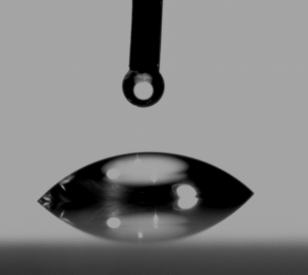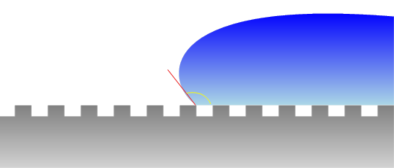## Contact Angles

### Quick Start

Simple ideas of contact angle measurement (see Surface Energy) are over-hyped. However, there are some interesting cases where the roughness of the surface has a significant effect on wetting, for example with Super-hydrophibic surfaces. And the dynamic contact angle is super-important for real-world coating.

Here we create some simple virtual structures and see what happens to the contact angle compared to the value θ on a plain surface. We define the surface with a fraction φ being solid and with a virtual depth. In the image below, φ=0.5 and Depth=1

We also see how the contact angle on a plain surface changes with speed, viscosity and surface tension.

### Contact Angles

Solid fraction φ
Depth 0-4
Contact Angle θ°
Type
"Roughness" r
Real angle θ*
Speed U m/min
Viscosity η cP
SurfTen σ mN/m
Contact Angle θ°
Ca
θdynamicIt is very easy to measure a static contact angle using modern automated methods with syringe needles and video cameras as shown in this image from Wikipedia. Unfortunately, these angles are almost useless because (usually) they are idealised angles on smooth substrates measured at low (or zero) velocities. The real contact angle depends both on surface structure at zero velocity and on viscosity and surface tension at higher speeds. This app calculates both angles independently (starting from different static contact angles). It does not calculate the velocity/dependent angle on a structured surface as that is just amassing complexities beyond the limits of both simple models.

The effect of surface structure is complex because there are three domains as defined by Quéré1:

1. Hemi-wicking - liquid "wicking" within the (rough) structure
2. Wenzel wetting - exaggeration of hydrophilic or hydrophobic effect
3. Super-hydrophobic - gross exaggeration of the hydrophobic effectGiven a surface of roughness r (ratio of actual surface area to what it would be if the plane were perfectly smooth) defined as being 1 for totally smooth to 10 being amazingly rough, and a fraction φ of that surface being solid and 1-φ being air then, for a static contact angle of θ on a flat surface the three contact angles θ* are given by:

Hemi-wicking: cos(θ"*") = φcos(θ)+1-φ

Wenzel: cos(θ"*") = 1+rcos(θ)

Super-hydrophobic: cos(θ"*") = -1+φ(cos(θ)+1)

Now we just need to know which domain we are in:

Hemi-wicking wins over Wenzel if: cos(θ) lt (1-φ)/(r-φ)

Super-hydrophibic wins over Wenzel if : cos(θ) lt (φ-1)/(r-φ)

So we need r and φ. This is quite complicated. For simplicity they are calculated from a rectangular structure of depth from 0 to 4 (relative to the width of the structure, with 4 being very hard to achieve) and the fractional width of the top, φ, from 0.01 (sharp spikes) to 1 (smooth surface).

The app works out which domain you are in and calculates θ*

The dynamic contact angle depends on the Capillary Number, Ca, which depends on velocity U, viscosity η and surface tension σ as

Ca = (Uη)/σ

The dynamic contact angle is then calculated using a very complicated equation from Shikhmurzaev. It is valid only for Ca<1. When Ca>=1 the value is highlighted in pink as a warning that the calculation is not likely to be accurate.

1David Quéré, Non-sticking drops Rep. Prog. Phys. 68 (2005) 2495–2532